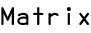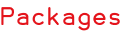RSS

Matrix represents an affine transformation between two coordinate spaces in 2 dimensions. Such a transform preserves the "straightness" and "parallelness" of lines. The transform is built from a sequence of translations, scales, flips, rotations, and shears.

The transformation can be represented using matrix math on a 3x3 array. Given (x, y), the transformation (x', y') can be found by:

```[ x']   [ scaleX shearX translateX ] [ x ]
[ y'] = [ shearY scaleY translateY ] [ y ]
[ 1 ]   [ 0      0      1          ] [ 1 ]

[ scaleX * x + shearX * y + translateX ]
= [ shearY * x + scaleY * y + translateY ]
[ 1                                    ]```

The bottom row of the matrix is constant, so a transform can be uniquely represented by "[[scaleX, shearX, translateX], [shearY, scaleY, translateY]]".